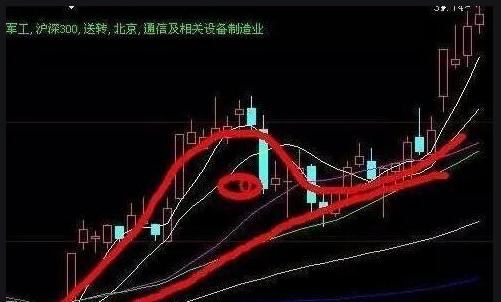# 老鸭头形态选股公式是什么？老鸭头形态选股公式详解

2023-05-09 16:35:38  |  来源:综合网络  |  编辑:  |A2:=(HHV(C,A1+1)/REF(C,A1+1)-1)*100;

A3:=CROSS(MA(C,5),MA(C,10));

A4:=LLV(V,10)=LLV(V,30)ANDEXIST(MA(V,5)>MA(V,10)ANDMA(V,10)>MA(V,20),30);

A5:=C/REF(C,A1+1)>1.05;

A2>10 AND A2MA(C,60);